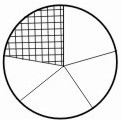HOME MATH DICTIONARY DOWNLOAD FEEDBACK DISCLAIMER
 Question: What are Fractions ? Answer: A fraction is part of a whole.For example, the given circle below has been split into 5 equal parts. Each is called a fifth, written 1 / 5. The fraction of the whole circle which has been crossed is: The number of crosed parts / The number of parts together = 1 / 5 (one fifths) The top number of a fraction is called the numerator and the bottom number is called denominator.